#### Chapter 18 Surface Area and Volume of a Cuboid and Cube R.D. Sharma Solutions for Class 9th Exercise 18.1

Exercise 18.1

1
Find the lateral surface area and total surface area of a cuboid of length 80 cm, breadth 40 cm and height 20 cm.

Solution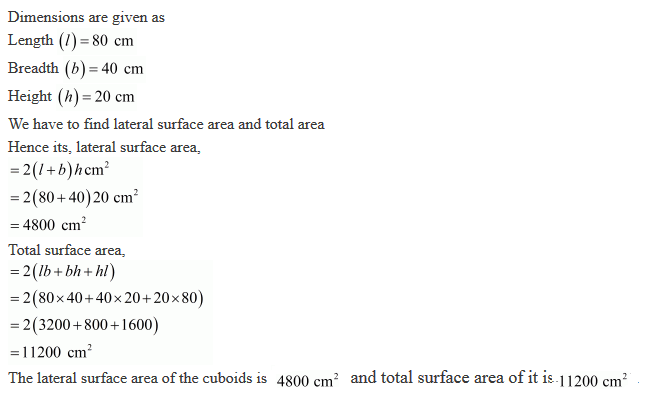2. Find the lateral surface area and total surface area of a cube of edge 10 cm.

Solution3. Find the ratio of the total surface area and lateral surface area of a cube.

Solution4. Marry wants to decorate her Christmas tree.. She wants to place the tree on a wooden block covered with coloured paper with picture of Santa Claus on it. She must know the exact quantity of paper to buy for this purpose. If the box has length, breadth and height as 80 cm, 40 cm and 20 cm respectively. How many square sheets of paper of side 40 cm would she require?

Solution5. The length, breadth and height of a room are 5 m, 4 m and 3 m respectively. Find the cost of white washing the walls of the room and the ceiling at the rate of Rs. 7.50 m2.

Solution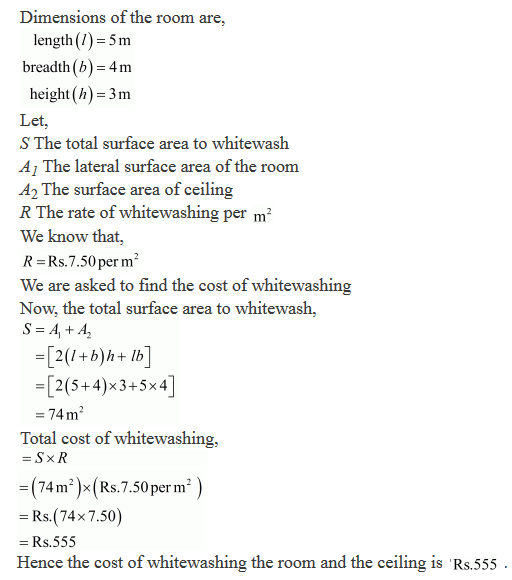6. Three equal cubes are placed adjacently in a row. Find the ratio of total surface area of the new cuboid to that of the sum of the surfaces areas of the three cubes.

Solution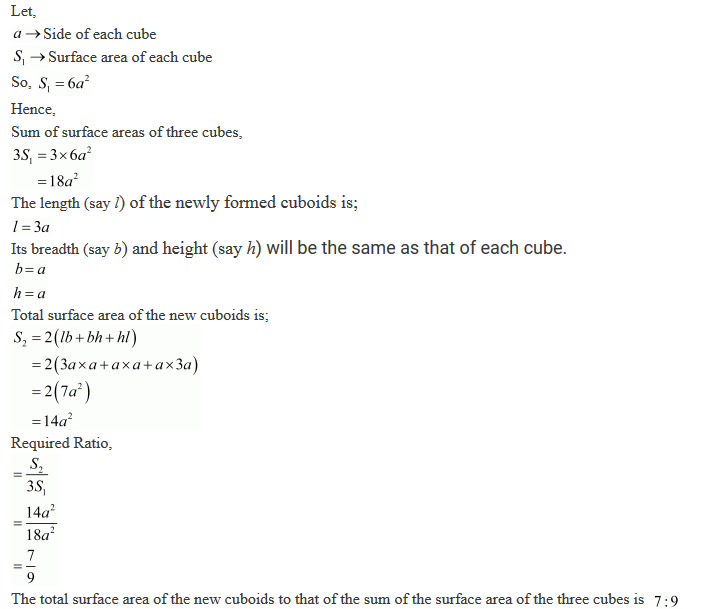7. A 4 cm cube is cut into 1 cm cubes. Calculate the total surface area of all the small cubes.

Solution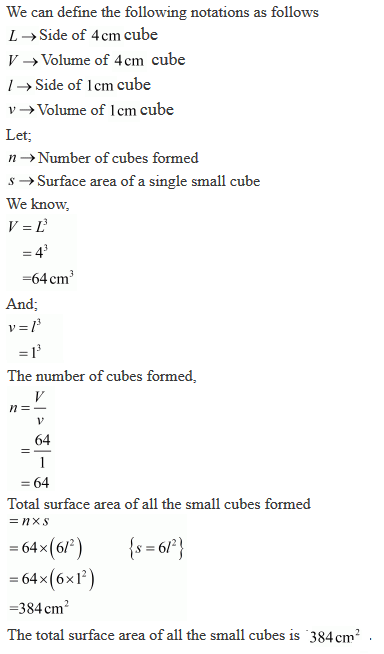8. The length of a hall is 18 m and the width 12 m. The sum of the areas of the floor and the flat roof is equal to the sum of the areas of the four walls. Find the height of the hall.

Solution9. Hameed has built a cubical water tank with lid for his house, with each other edge 1.5 m long. He gets the outer surface of the tank excluding the base, covered with square tiles of side 25 cm. Find how much he would spend for his tiles, if the cost of tiles is Rs 360 per dozen.

Solution10. Each edge of a cube is increased by 50%. Find the percentage increase in the surface area of the cube.

Solution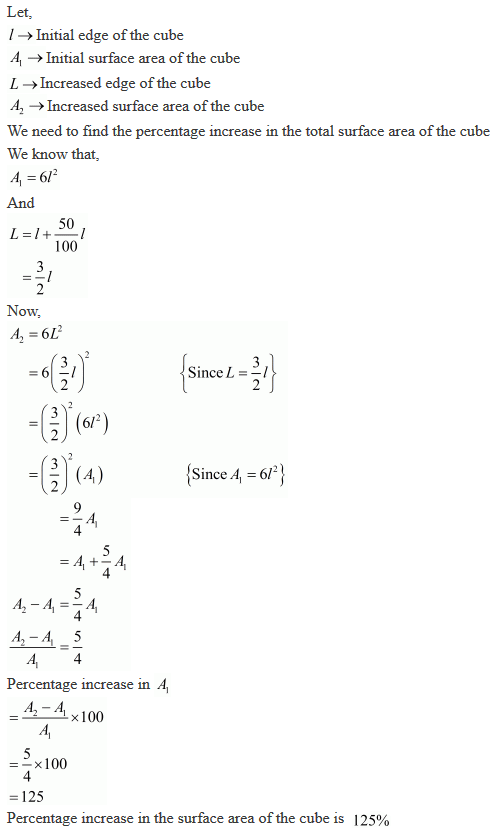11. The dimensions of a rectangular box are in the ratio of 2 : 4 and the difference between the cost of covering it with sheet of paper at the rates of Rs 8 and Rs 9.50 per m2 is Rs 1248. Find the dimensions of the box.

Solution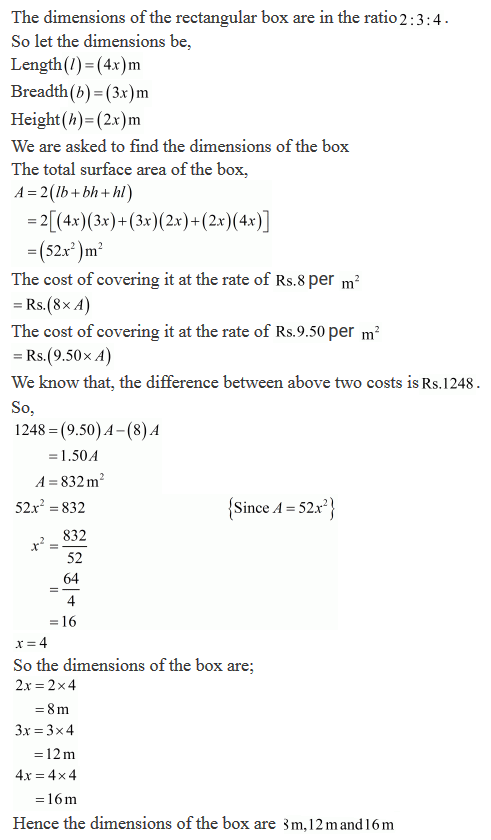12. A closed iron tank 12 m long, 9 m wide and 4 m deep is to be made. Determine the cost of iron sheet used at the rate of Rs 5 per metre sheet, sheet being 2 m wide.

Solution13. Ravish wanted to make a temporary shelter for his car by making a box-like structure with tarpaulin that covers al the four sides and the top of the car (with the front face as flap which can be rolled up). Assuming that the stitching margins are very small, and therefore negligible, how much tarpaulin would be required to make the shelter of height 2.5 m witt base dimensions 4 m ✕ 3 m ?

Solution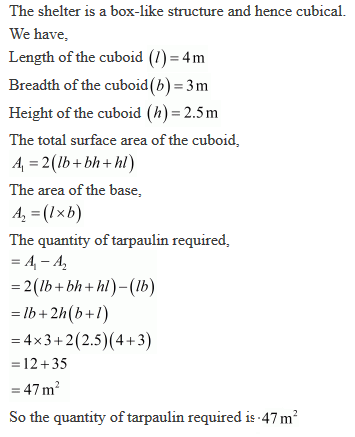14. An open box is made of wood 3 cm thick, its external length, breadth and height are 1.48 m, 1.16 m and 8.3 dm. Find the cost of painting the inner surface of Rs 50 per sq. metre.

Solution15. The cost of preparing the walls of a room 12 m long at the rate of Rs 1.35 per square metre is Rs 340.20 and the cost of matting the floor at 85 paise per square metre is Rs. 91.80. Find the height of the room.

Solution16. The dimensions of a room are 12.5 m by 9 m by 7 m . There are 2 doors and 4 windows in the room; each door measures 2.5 m by 1.2 m and each window 1.5 m by 1 m . Find the cost of painting the walls at Rs 3.50 per square metre.

Solution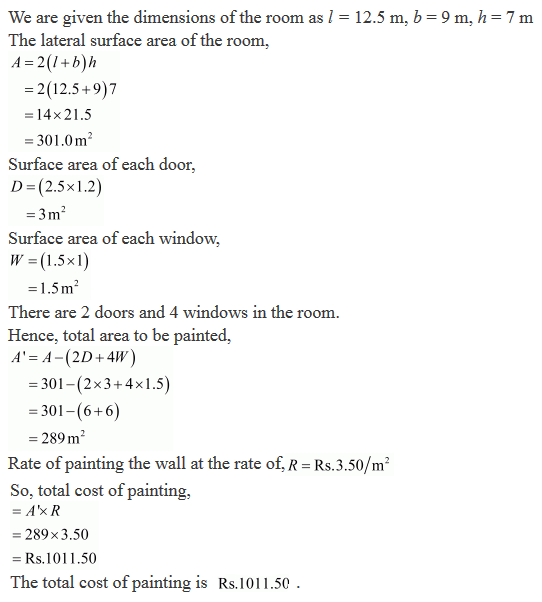17. The length and breadth of a hall are in the ratio 4 : 3 and its height is 5.5 metres. The cost of decorating its walls (including doors and windows) at Rs 6.60 per square metre is Rs 5082. Find the length and breadth of the room.

Solution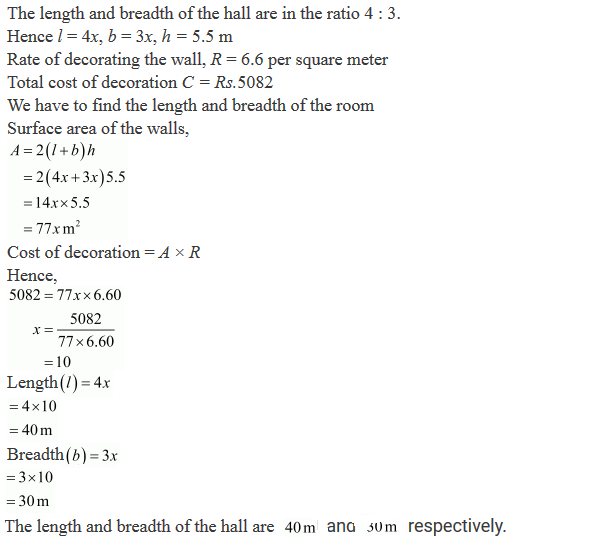18. A wooden bookshelf has external dimensions as follows: Height = 110 cm, Depth = 25 cm, Breadth = 85 cm (See Fig. 18.5). The thickness of the plank is 5 cm every where. The external faces are to be polished and the inner faces are to be painted. If the rate of polishing is 20 paise per cm2 and the rate of painting is 10 paise per cm2. Find the total expenses required for polishing and painting the surface of the bookshelf.Solution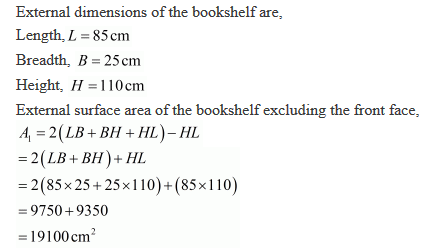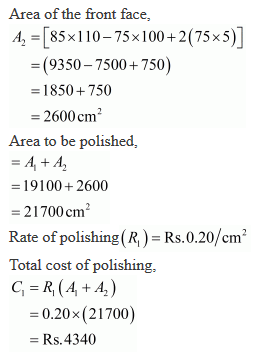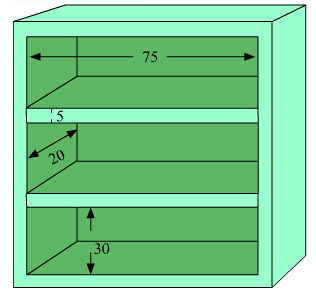19. The paint in a certain container is sufficient to paint on area equal to 9.375 m2. How many bricks of dimension 22.5 cm × 10 cm × 7.5 cm can be painted out of this container?

Solution